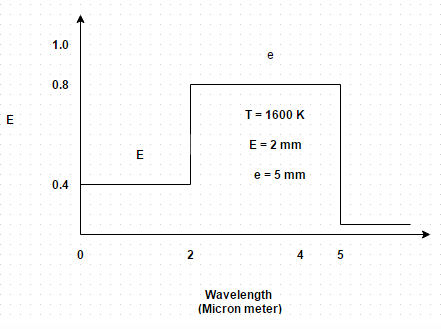# Test: Gray Body And Selective Emitters

## 10 Questions MCQ Test Heat Transfer for Engg. | Test: Gray Body And Selective Emitters

Description
This mock test of Test: Gray Body And Selective Emitters for Chemical Engineering helps you for every Chemical Engineering entrance exam. This contains 10 Multiple Choice Questions for Chemical Engineering Test: Gray Body And Selective Emitters (mcq) to study with solutions a complete question bank. The solved questions answers in this Test: Gray Body And Selective Emitters quiz give you a good mix of easy questions and tough questions. Chemical Engineering students definitely take this Test: Gray Body And Selective Emitters exercise for a better result in the exam. You can find other Test: Gray Body And Selective Emitters extra questions, long questions & short questions for Chemical Engineering on EduRev as well by searching above.
QUESTION: 1

### When Stefan-Boltzmann law is applied to a black body, it takes the form

Solution:

The constant is different for different bodies.

QUESTION: 2

### Consider two bodies, one absolutely back and the other non-black and let these be at same temperature. Which one of the following statement is correct?

Solution:

The radiation spectrum for a non-black body may be similar or radically different from that of a black body.

QUESTION: 3

### When the emissivity of non-black surface is constant at all temperatures and throughout the entire range of wavelength, the surface is called

Solution:

The radiation spectrum for a grey body, though reduced in vertical scale, is continuous and identical to the corresponding curve for a perfectly black surface.

QUESTION: 4

The emissivity of the gray surface may be expressed as

Solution:

It is the ratio of radiating coefficient to that of black body radiating coefficient.

QUESTION: 5

If a black body at 1000 K and a gray body at 1250 K emit the same amount of radiation, what should be the emissivity of the gray body?

Solution:

E = (T b/T G) 4 = 0.4096.

QUESTION: 6

The radiant heat transfer from a plate of 2.5 cm2 area at 1250 K to a very cold enclosure is 5.0 W. Determine the emissivity of the plate at this temperature

Solution:

Emissivity = E/σ A T4 = 0.144.

QUESTION: 7

A 100 W light bulb has a tungsten filament (emissivity = 0.30) which is required to operate at 2780 K. If the bulb is completely evacuated, calculate the minimum surface area of the tungsten filament

Solution:

E = (Emissivity) σ A T4. So, A = 0.98 * 10 -4 m2.

QUESTION: 8

The monochromatic emissivity € of a diffuse surface at 1600 K varies with wavelength in the following manner
€ = 0.4 for 0 < λ < 2
= 0.8 for 2 < λ < 5
Determine the total emissivitySolution:

Total emissivity = 0.4 (-0.3181 – 0.0000) + 0.8 (0.8563 – 0.3118) = 0.5578.

QUESTION: 9

For a hemisphere solid angle is measured as

Solution:

It should be measured in Steradian.

QUESTION: 10

A gray body (E = 0.8) emits the same amount of heat as a black body at 1075 K. Find out the required temperature of the gray body

Solution:

b= E T g4.